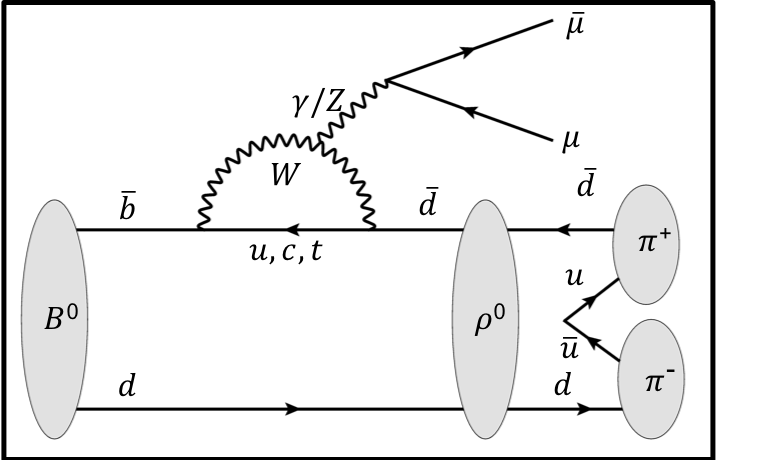# Edward Millard

I am a PhD student working on the LHCb experiment studying the rare decays of B hadrons.

I am currently working on an analysis of the rare decay$B^0\rightarrow\pi^+\pi^-\mu^+\mu^-$ which is an example of a b$\rightarrow$ d flavour changing neutral current transition.

I am responsible for helping check new DecFile releases as part of the GAUSS project.

I also teach first year undergraduate physics problems classes.

The Standard Model of particle physics, despite its many successes, is known to be imperfect. Many particle physics experiments, including LHCb, test the standard model and measure its parameters in the hope of finding evidence of new physics.

New physics could potentially influence the decays of certain b and c hadrons by contributing as virtual particles at loop level. b$\rightarrow$ s processes (an example of a flavour changing neutral current) can only occur at loop level in the standard model and are very rare. Therefore any new physics contribution to these processes would have a comparatively large effect making them identifiable.

There are currently exciting tensions seen by LHCb with the Standard Model in certain b$\rightarrow$ s processes. These include, for example, the angular distribution and decay rate of the$B^0\rightarrow K^{*0}\mu^+\mu^-$ decay. The motivation Is therefore there to study the analogous b$\rightarrow$ d processes which are even more suppressed and may provide further hints at the possibility of new physics.

An example of a b$\rightarrow$d process is$B^0\rightarrow\rho^{0}\mu^+\mu^-$ where$\rho^0\rightarrow\pi^+\pi^-$. This is the current focus of my research. The aim of my project is to complete a branching fraction analysis and an angular analysis of this decay.Feynman diagram of the rare decay$B^0 \rightarrow\rho^{0}\mu^+ \mu^-$ with$\rho^0\rightarrow\pi^+\pi^-$

##### Contact Details

Office: P449

e-mail: E.J.Millard@warwick.ac.uk